# Homework Help Question and Answers

## Question 44981

posted 10 months ago

## -f z=m\left(\frac{d^{2} z}{d t^{2}}\right)O What is the mathematical operator in this equation?(10%) Under what conditions is the function z(t) = B sin (wt) a solution of Eq. 6.2? Justify your answer by showing a detailed proof.## Question 44980

posted 10 months ago

## Q2 (40%) Consider a system at 400 K which has an infinite ladder of evenlyspaced quantum states with an energy spacing of o.15 kJ/mol. You may assumethat the ground state has an energy of ɛo= o and that all the states are non-degenerate (g = 1).1. (10%) What is the minimum possible value of the partition function for this system? JustifyO What is the value of BAɛ for this system?3. (10%) Estimate the number of quantum states which are populated.thermally4. (10%) If the temperature is doubled, estimate the number of thermally populated states.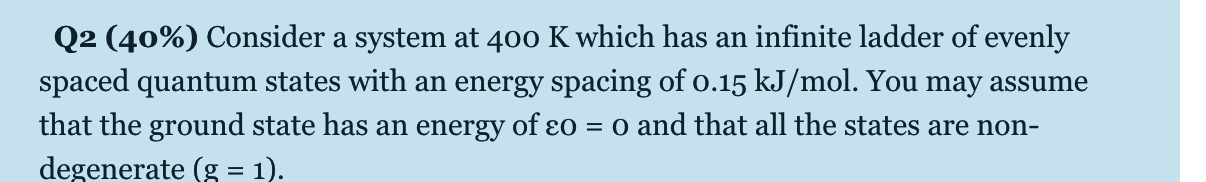## Question 44979

posted 10 months ago

## Q1 (40%) Given that translational and rotational degrees of freedom typically behave classically, but vibrations behave quantum mechanically, the total(average) energy of a diatomic is predicted to be, U = (5/2)RT + <e>, where <e> is obtained from Eq. 1.24 in the textbook.1. (10%) Calculate the contribution from translation and rotation to the molar heat capacity of the Cl2 gas at 400 K.2. (10 %) Calculate the contribution from vibration to the molar heat capacity of the Cl2 gas at 400 K, given that diatomic molecule Cl, has a vibrational wavenumber of 565 cm¬1.3. (10%) Predict the molar heat capacity of Cl2 gas at 400 K and compare your result with the experimental molar heat capacity of Cl2 , Cy × 29 J/(K mol) at400 K.6) Calculate the average internal energy <U> for the Cl2 gas at 400 K.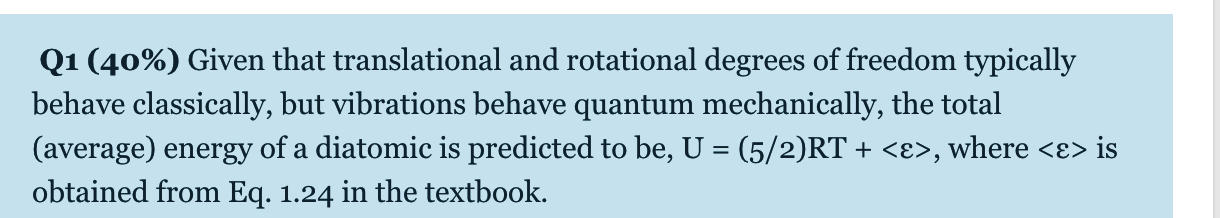## Question 38933

posted 11 months ago

## For a Na*-Cl ion pair, attractive and repulsive energies EA and ER, respectively, depend on the distance between the ions r, according toE_{A}=-\frac{1.436}{r} \quad \text { and } \quad E_{R}=\frac{7.32 \times 10^{-6}}{r^{8}}For these expressions, energies are expressed in electron volts (eV) per Na*–Cl¯ pair, and r is-the distance in nanometers (nm). The net energy En is just the sum of the preceding two expressions.(a) Superimpose on a single plot EN, ER, and EA versus r up to 1.0 nm.(b) On the basis of this plot, determine (i) the equilibrium spacing ro between the Na* and Cl-ions, and (ii) the magnitude of the bonding energy Eo between the two ions.## Question 38934

posted 11 months ago

## 4. Briefly cite the main differences between ionic, covalent, and metallic bonding.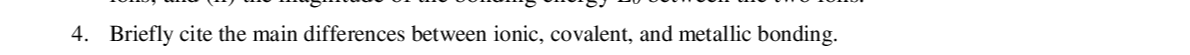## Question 38936

posted 11 months ago

## 6. One material normally has multiple types of bonding. Some are dominant (major) bonding and some are non-dominant (minor) bonding. What type(s) of bonding would be expected for each of the following materials: solid xenon, calcium fluoride (CaF2), bronze (a copper-tin alloy), cadmiumtelluride (CdTe), rubber, and tungsten? Please identify the major and the minor types of bonding.## Question 38931

posted 11 months ago

## 1. Give the corresponding electron configurations for following element atoms, their valence electrons,and their ions, respectively. (Please note the differences in electron arrangements for neutral atoms and ions)## Question 38932

posted 11 months ago

## (a) Schematically plot the curve of net potential energy (En) vs. interatomic separation (r) for two isolated atoms. Indicate on the plot the equilibrium separation ro and the bonding energyEọ.(b) Schematically plot the curve of net force (FN) vs. interatomic separation (r) for two isolated atoms. Indicate on the plot the equilibrium separation ro.(c) Briefly describe the behaviors of the net potential energy and the net force at the vicinity of equilibrium separation ro, i.e. how the energy and force change with the change of interatomic separation around ro.## Question 38935

posted 11 months ago

## 5. Compute the percentage ionic character of the interatomic bond for GaP and CsF.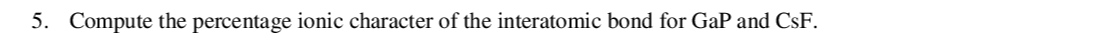## Question 40360

posted 1 years ago

## (a) Starting from the definition of an acid dissociation constant, derive an equation that relates the pH of an equilibrium mixture of an acid and its conjugate base to the composition of the solution.(b) Which ionisable groups are likely to contribute to the buffering capacity of the intracellular medium at normal intracellular pH values? What other mechanisms are available for maintaining intracellular pH values at appropriate values?(c) The pKa of methanoic acid is 3.8. What ratio of methanoic acid to sodium methanoate would be necessary to give a solution of pH 4.5?(d) Methanoic acid/methanoate is going to be used as a buffer for a reaction. The unbuffered reaction at pH 4.5 results in a decrease in the pH of 2.5 units. What is the minimum concentration of the buffer needed to prevent the pH falling below 4.25?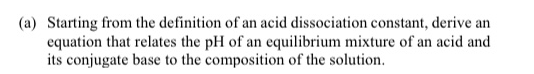## Most popular subject### Thermodynamics### Essay/Summary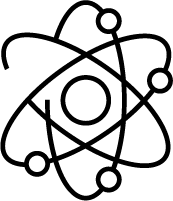### Mechanics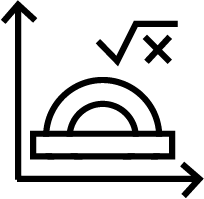### Complex Analysis### Engineering Economics### Calculus### Modern Physics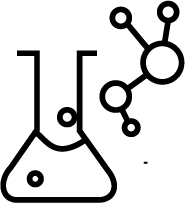### General Chemistry### Strength Of Materialsx